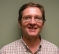## Sigmoid functionStarted by 10 months ago8 replieslatest reply 10 months ago324 views

Hello I am looking to implement a sigmoid function as an activation function for ML(I do know that there is now a ML Related). I need to implement this is a fixed point fashion with a 16 bit signed input, likely something like Q2.13 to Q3.12 and an output of Q0.15.

For those who are unfamiliar with a sigmoid the function is given by:

Sigmoid(x) = 1 / (1 + exp(-x))

Alternatively: https://en.wikipedia.org/wiki/Sigmoid_function

Any recommendations or tricks I can use would be greatly appreciated.

[ - ]It looks like you are trying to classify several things into categories. What problem are you trying to solve? This is usually an output function to show the probability of the classification.

[ - ]The problem I am trying to solve is to implement a sigmoid function as part of a library intended to be used to implement ML graphs. It is there fore reasonable for me to assume an input to the sigmoid in the range of [-6, +6] represented by 16 bits and an output between [0, 1] at 16 or 8 bits.

[ - ]Think I would 2nd the suggestion of a look up table.  I would also look at interpolation to keep the table size reasonable.  See if there isn't something like a taylor approximation or some other series function that could be used as an interpolation between points.

[ - ]Do you actually need LUT if you use interpolation ?

Here's an example, I made, of approximation of the Sigmoid -function using Krogh's interpolation algorithm:

There 4-point interpolation is done for equally spaced points in range [0,6].
y = f(|x|)           | x {0, 6}
y = 1 - f(|x|)       | x {-6, 0} 

Dunno if this type of method is too slow when coded using ML language (OP maybe don't want to use exp(), I assume math is not well handled by ML compiler).

With different location of reference points and different interpolation method one could try improve the accuracy and also simplify the calculation process.

Example: Approximate Sigmoid -function using polynomial got from 4-point Neville's interpolation algorithm: https://www.desmos.com/calculator/5pu0qnel2a

[ - ]I would use a look up table with interpolation. If you have the space you can save the interpolation and just go for a full 64k table.

[ - ]16 bits signed output requires +32k/-32K lut.

lut can be reduced to +/-16k or less if resolution ok.

There is also anti-symmetry if offset added.

For 8 bit output you need small lut anyway

[ - ]Hi, a combination of LUT with newton-raphson will help.

the basic idea is keep a very course LUT. then increment the xr by small factor (dx) to reach the actual x. then find yr.

y(n+1) = y(n) + y'[n]*dx

dy = the dy/dx ay point xr. for sigmoid it is =  yr * (1 - yr)

so the C code will look some like this :

        idx = floor(x) - GSA_LUT_MATH_SOFT_CLIP_MIN - 1;
yr = gsa_lut_math_soft_clip[idx].yr;    // y0
dy = gsa_lut_math_soft_clip[idx].dy;    // y'
xr = florr(x) - 1.0f;
while (xr < x)
{
yr += dy * dx;
dy = yr * (1 - yr);
xr += dx;
}
y = yr;



hope it helps.

Chalil

[ - ]